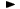ADVFN HomeHelpFinancialsDeeper AnalysisFINANCIAL RATIOS - Liquidity RatiosQuick Ratio (Acid Test)

# Quick Ratio (Acid Test)

Quick ratio (or Acid Test), expresses a company's ability to repay short-term creditors out of its most liquid assets. The quick ratio is the result of dividing the total of current assets, other than stocks, by short-term borrowings. It shows the number of times short-term liabilities are covered by quick assets. If the value is greater than 1.00, it means fully covered.

The formula is as follows:

= (debtors + cash & equivalents + current assets,other) / creditors,short